# Prime divisor of Fermat number is congruent to one modulo large power of two

## Statement

Suppose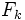is theFermat number, i.e.,. Then, we have:

• Ifis a prime divisor of, then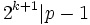.
• For,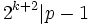.

## Proof

Given: A nonnegative integer.is theFermat number.is a prime divisor of.

To prove:divides, and,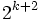divides.

Proof: Consider the order ofmodulo. We have: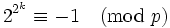.

Thus:.

The order ofmodulodividesbut does not divide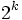. Hence, it equals.

On the other hand, by Fermat's little theorem, the order ofmodulodivides. Thus,divides.

Now consider the case. In this case,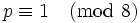, and thus, by fact (1),is a quadratic residue modulo. Thus, there existssuch that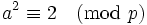. This forces that the order ofmodulois. Combining this with Fermat's little theorem, we obtain thatdivides.